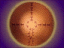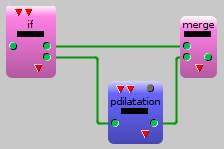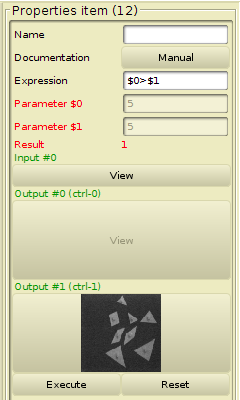ARIANE: A Visual Programming Interface

# The Control Structure: If

## Definition

A control structure If is an item which implements alternative. The result of a boolean expression determines the index of the output slot between two. If the value is true then the first output is used, otherwise the second. Generally, it is necessary to use a component merge to recombine the two alternative paths into one such as in the following figure:## Properties### Parameters

This items has two parameters named respectively:

• >\$0 the first parameter
• \$1 the second parameter

Alternative is related to the following instruction:

```   if (test between \$0 and \$1) do
then
copy input into output 0;
else
copy input into output 1;
```

### Test Expression

The test is a boolean expression built with conjunction, disjunction or negation connectors between constants or values of the two parameters. More complex expressions should be formulated with the eval item.

Regular boolean expressions must be built with:

• <
• >
• <=
• >=
• ==
• !=

and the logical connectors:

• & (AND)
• | (OR)
• ^ (XOR)
• ! (NOT)

#### Example of valid test

`(\$0>3.0) | (\$1<=5)`

### Result value

The item execution returns the validated output index: 0 or 1.